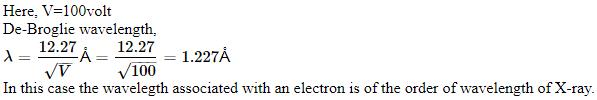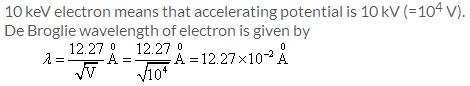Courses

# Test: Dual Nature Of Matter

## 10 Questions MCQ Test Physics For JEE | Test: Dual Nature Of Matter

Description
This mock test of Test: Dual Nature Of Matter for JEE helps you for every JEE entrance exam. This contains 10 Multiple Choice Questions for JEE Test: Dual Nature Of Matter (mcq) to study with solutions a complete question bank. The solved questions answers in this Test: Dual Nature Of Matter quiz give you a good mix of easy questions and tough questions. JEE students definitely take this Test: Dual Nature Of Matter exercise for a better result in the exam. You can find other Test: Dual Nature Of Matter extra questions, long questions & short questions for JEE on EduRev as well by searching above.
QUESTION: 1

### What is the de-Broglie wavelength associated with an electron accelerated through a potential of 100 volts?

Solution:and as we know 1Å = 0.1 nm, so 1.227Å = 0.122 nm

QUESTION: 2

### Davisson and Germer experiment proves the

Solution:

The Davisson-Germer experiment demonstrated the wave nature of the electron, confirming the earlier hypothesis of deBroglie. Putting wave-particle duality on a firm experimental footing, it represented a major step forward in the development of quantum mechanics. The Bragg law for diffraction had been applied to x-ray diffraction, but this was the first application to particle waves.

QUESTION: 3

### An electron, proton and a jeep all have the same wavelength. The one possessing the highest velocity is​

Solution:

The de-broglie equation states, mv=h/l

= v=h/lm

the velocity here is inversely dependant on the masses.

Higher the mass lower will be the velocity.

Therefore, electron will have a higher velocity to produce matter waves of same wavelength.

QUESTION: 4

Objects with mass behave as

Solution:

We have mass – a particle property, in the same equation as wavelength – a wave property. Thus if matter exhibits wave properties, it must be given by equation (3). Equation (3) is the de Broglie equation and represents the wave-particle duality. Hence we say that everything in the Cosmos exhibits a dual nature.

QUESTION: 5

An α – particle and a deutron are accelerated through the same potential difference. What will be the ratio of their de-Broglie wavelength?​

Solution:

Mass of alpha(a)= 4.
mass of deutron(d) =2.
wavelength=h/mv
Potential are same of both particle so their speed will be same .
Here, wavelength is inversely proportional to mass
so,
wavelength of alpha/wavelength of deutron = mass of deutron /mass of alpha.= 2/4=1/2

QUESTION: 6

Of the following moving with the same momentum, the one which has the largest wavelength is​

Solution:

De Broglie wavelength = h/mv,
where h = plancks constant, mv = momentum.
As they move with same momentum, de Broglie wavelength remains constant for all.

QUESTION: 7

The velocity of matter waves are​

Solution:

The variable v can either be taken to be the speed of the particle or the group velocity of the corresponding matter wave. Since the particle speed for any particle that has mass (according to special relativity), the phase velocity of matter waves always exceeds c, i.e.

QUESTION: 8

The wavelength of 1 keV photon is

Solution:

There is a direct relation ,
energy= 12400/wavelength(i e ,lambda in angstroms).
given E= 1k e v = 1000 e.v
wavelength = 12400/1000
wavelength= 124/10
wavelength= 12.4

QUESTION: 9

The de-Broglie wavelength of an electron is 1.0 nm. What is the retarding potential required to stop it?​

Solution:

λ=h/P
Here P= √2mk
K=kinetic energy
λ=h/√2mk
By energy conversion
K=eVs
λ=h/√2meVs
√Vs=h/ λ√2me
Vs=h2/ λ2me
Vs=(6.626x10-34)2/(1x10-9)2x2x9.11x10-31xe
Where e=1.602x10-19
Vs=43.9x10-68/29.1x10-68
=1.508v
Vs=1.5v

QUESTION: 10

The de Broglie wavelength of 10 KeV electron is

Solution: